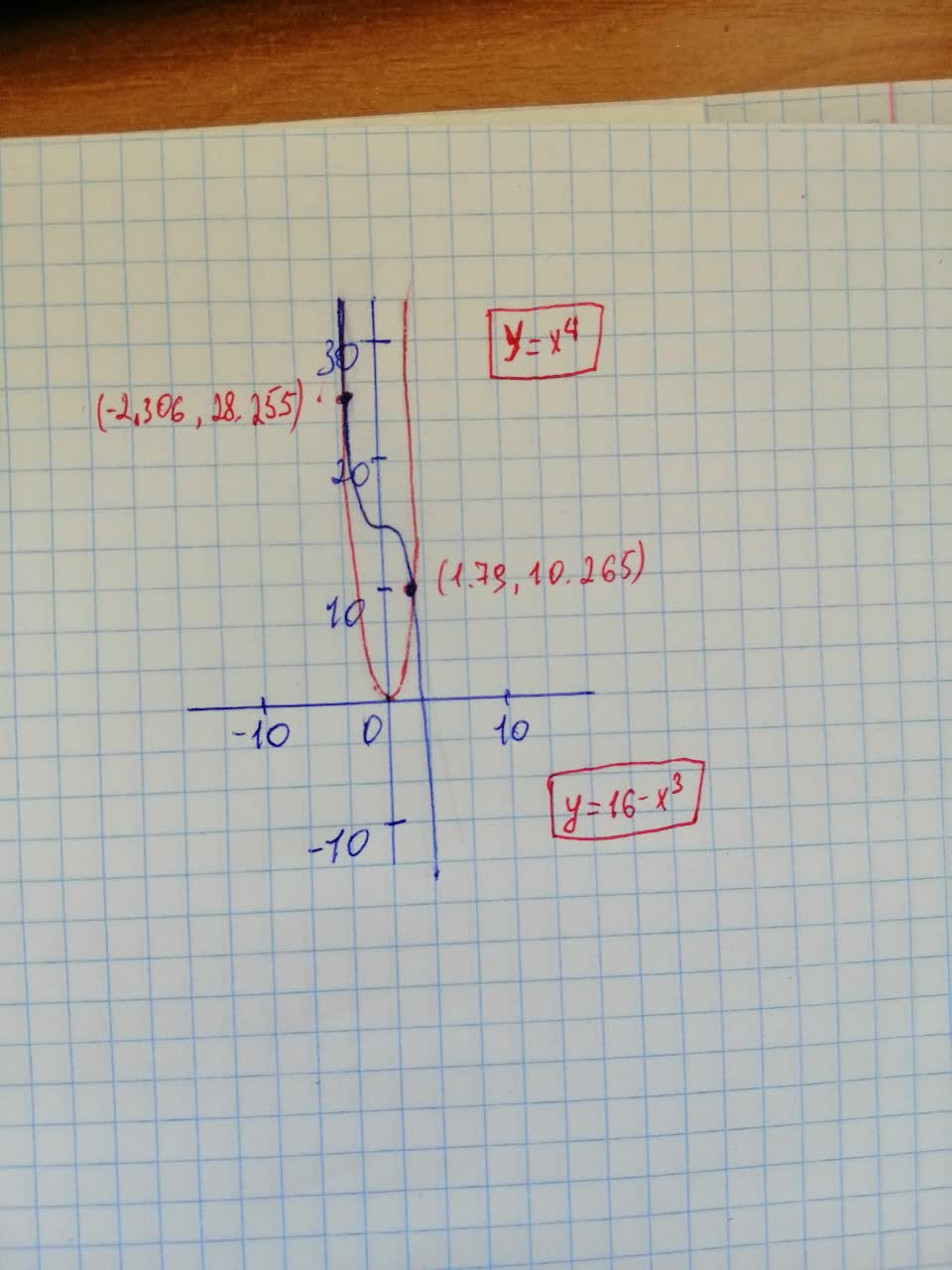Question# Find all real solutions of the equation X^{4}=16-x^{3}, rounded to two decimals.

Decimals
ANSWERED$$\displaystyle{X}^{{{4}}}={16}-{x}^{{{3}}}$$ - Find all real solutions of the equation, rounded to two decimals.2021-08-10
Step 1
Given equation is
$$\displaystyle{x}^{{{4}}}={16}-{x}^{{{3}}}$$
We need to find all the real solutions, rounded to two decimals.
Step 2
Since there are no easy roots for the equation, we find the real roots graphically.
We plot $$\displaystyle{y}={x}^{{{4}}}$$ and $$\displaystyle{y}={16}-{x}^{{{3}}}$$ and we graphically calculate the intersection points of these two curves.Roots are
$$\displaystyle{x}_{{{1}}}={2.31}$$
$$\displaystyle{x}_{{{2}}}={1.79}$$